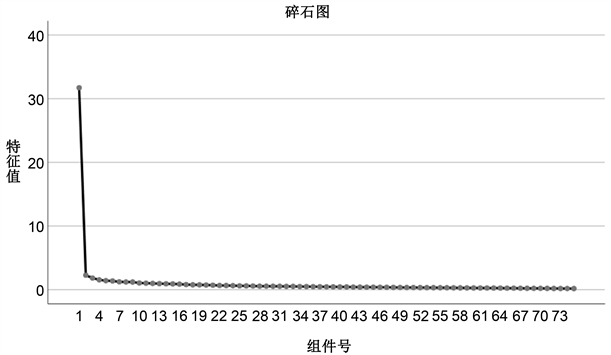﻿ 基于主成分分析的青年军人心理健康状况评估及应对措施研究

# 基于主成分分析的青年军人心理健康状况评估及应对措施研究Research on Mental Health Status Assessment and Countermeasuresof Young Soldiers Based on Principal Component Analysis

Abstract: This article is based on 1264 SCL-90 scale test questionnaires filled out by young soldiers. Using the principal component analysis method, the six most significant factors in the mental health problems of young soldiers are terror anxiety, coercion, nervousness, poor oxygen supply, physical fatigue and hostility. Combined with qualitative analysis, the six factors can be organized into four aspects: fear anxiety, coercion, physical discomfort and hostility, and then countermeasures are proposed for these four aspects, which has certain enlightening significance for adapting the mental state of military personnel.

1. 引言

2. 问卷的采集与处理

${y}_{i}=\frac{{x}_{i}-\stackrel{¯}{x}}{s}$ (1)

3. 青年军人心理健康状况的主成分分析

1) 对原始指标进行标准化处理；

2) 考察指标间的相关性，改善指标间存在的多重共线性；

3) 对改善后的指标进行主成分分析。

3.1. 运用R聚类改善指标间的多重共线性

1) 根据实际情况，我们设定聚类阈值为0.6，指标间相关系数高于0.6，则可聚为一类；

2) 计算各类中问题指标的均方相关系数，取最大者为该类代表；

3) 去掉各类中除代表指标以外的其余问题指标。

${r}_{ij}=\frac{\underset{k=1}{\overset{n}{\sum }}\left({x}_{ki}-{\stackrel{¯}{X}}_{I}\right)\left({x}_{kj}-{\stackrel{¯}{X}}_{j}\right)}{{\left(\underset{k=1}{\overset{n}{\sum }}{\left({x}_{ki}-{\stackrel{¯}{X}}_{I}\right)}^{2}\underset{k=1}{\overset{n}{\sum }}{\left({x}_{kj}-{\stackrel{¯}{X}}_{j}\right)}^{2}\right)}^{\frac{1}{2}}}$ (2)

$D\left(p,q\right)=\frac{1}{{n}_{p}{n}_{q}}\underset{{x}_{i}\in {G}_{p}}{\sum }\underset{{x}_{j}\in {G}_{q}}{\sum }{r}_{ij}$ (3)

${\stackrel{¯}{R}}_{i}=\sqrt{\frac{1}{k-1}\underset{j\ne i}{\sum }{r}_{i\text{ }j}^{2}}$ (4)Table 2. Clustering item index correlation table ( r i j > 0.6 )Table 3. Selection process of various representative indicators

3.2. 对于改善后问题指标的主成分分析

1) 求标准化问题指标的相关系数矩阵 ${R}_{ij}$，并对其进行KMO检验和Bartlett球形检验；

2) 求实特征值 ${\lambda }_{1},\text{\hspace{0.17em}}{\lambda }_{2},\text{\hspace{0.17em}}\cdots ,\text{\hspace{0.17em}}{\lambda }_{p}$，及对应的p个标准特征向量；

3) 根据特征值 ${\lambda }_{1}$ 的大小排序，结合方差累计贡献率和 ${\lambda }_{1}$ 的变化特征确定主成分个数；

4) 根据主成分因子负荷大小解释主成分。

${R}_{ij}={\left(\begin{array}{cccc}1& 0.352& \cdots & 0.245\\ 0.352& 1& \cdots & 0.405\\ \cdots & \cdots & \cdots & \cdots \\ 0.245& 0.405& \cdots & 1\end{array}\right)}_{75×75}$ (5)Table 4. Cumulative contribution rate of varianceFigure 1. Eigenvalue lithotripsyTable 5. Factor load table (75 × 6)

4. 青年军人心理问题的主成分因子Table 6. Main components of mental health problems of young soldiers

5. 加强青年军人心理调适的建议措施

5.1. 构建三级援助模式，加强官兵心理疏导

5.2. 加强普及心理知识，提高官兵心理认知

5.3. 及时跟进心理干预，抑制心理问题生长

5.4. 密切协调人际关系，构建集体和谐氛围

5.5. 提高心理训练质量，增强心理调适能力

 陈继军, 吴雪冰, 刘清波(2015). 用SCL90、EPQ调查某部队神经症结果分析. 健康心理学杂志, 8(1), 112-114.

 冯正直, 胡丰, 刘云波, 等(2016). 我国军人症状自评量表2016版常模的建立. 第三军医大学学报, 38(20), 2210-2214.

 何晓群(2015). 多元统计分析(第四版). 北京: 中国人民大学出版社.

 姜锐(2016). 重庆某部队战士心理健康与体能相关性分析. 中国卫生产业, 13(18), 25-27.

 李国华(2017). 战士的心理成因及对策军事. 心理学杂志, 14(1), 46.

 廖雅琴, 胡彦述, 冯正直(2005). 我国军人心理健康研究的现状与展望. 第三军医大学学报, (20), 2090-2092.

 衣新发, 赵倩, 蔡曙山(2012). 中国军人心理健康状况的横断历史研究: 1990-2007. 心理学报, 44(2), 226-236.

 张文彤(2013). SPSS统计分析高级教程(第二版). 北京: 高等教育出版社.

 张小战, 朱爱荣, 唐华(2016). 某部官兵心理健康状况调查解放军. 預防医学杂志, 21(1), 27-29.

 Dew, M. A., Soria, J. J., & Seghezzo, M. E. (1997). Validity and Reliability of Symptom Checklist 90(SCL-90) in an Argentine Bonicatto Population Sample. Social Psychiatry and Psychiatric Epidemiology, 32, 332-338.
https://doi.org/10.1007/BF00805438

 Holi, M. (2003). Assessment of Psychiatric Symptoms Using the SCL-90. Academic Dissertation, Helsinki: The University of Helsinki.

Top